# Lectures synchronous machines(1)

Oct. 1, 2016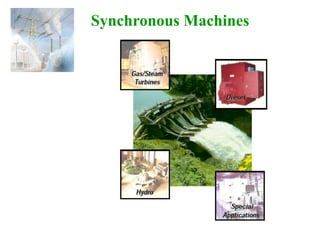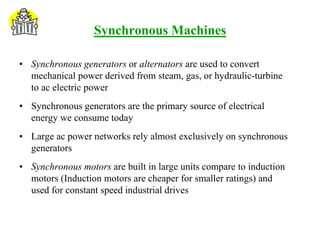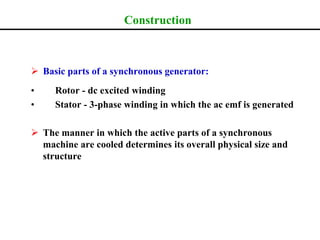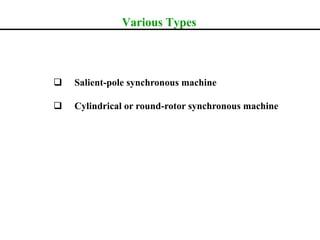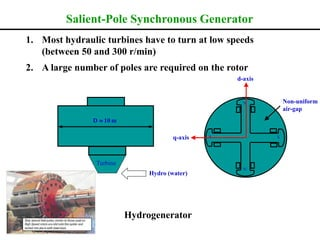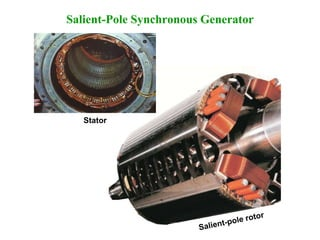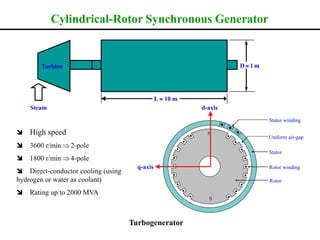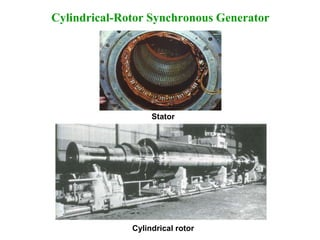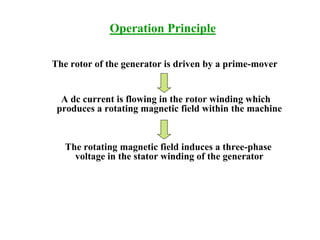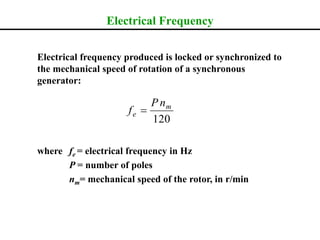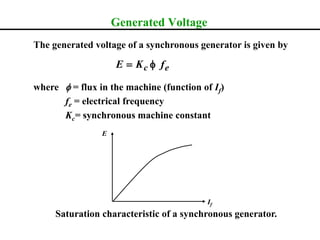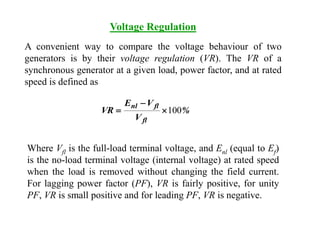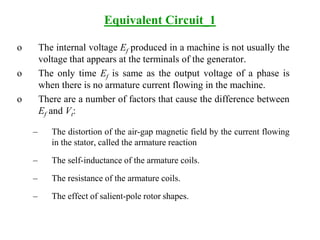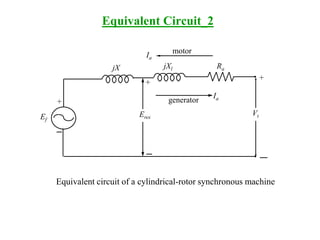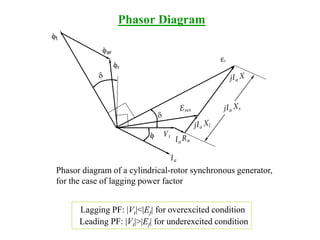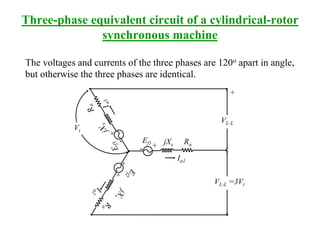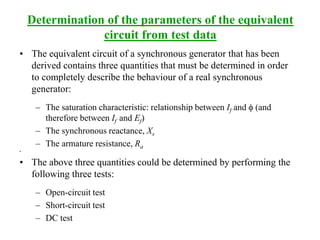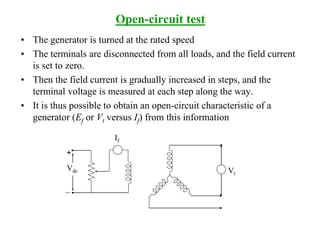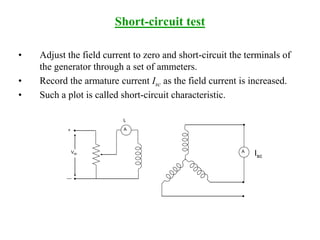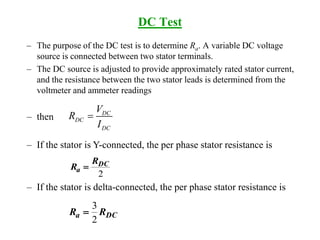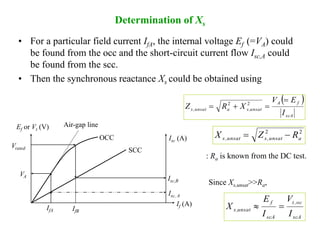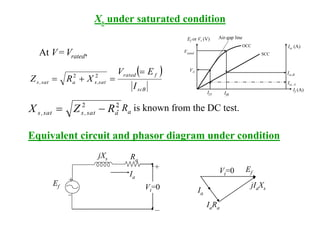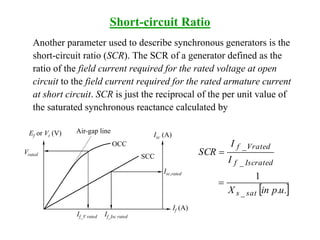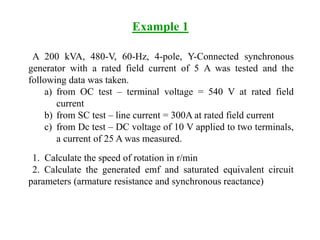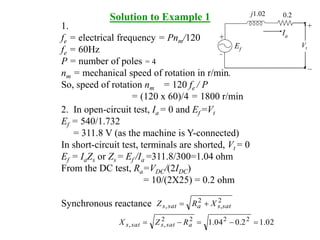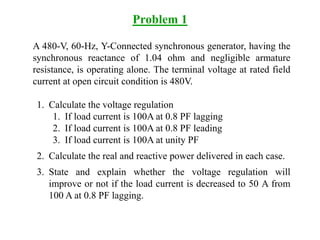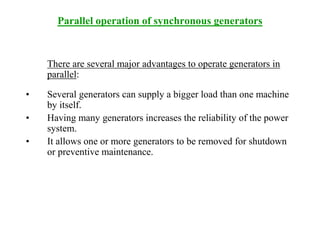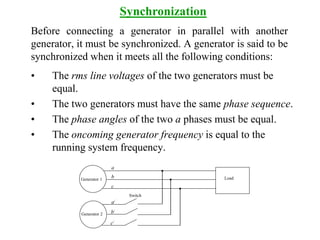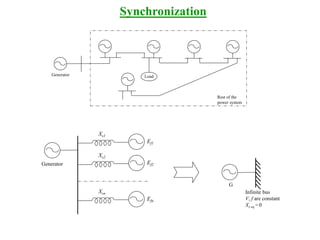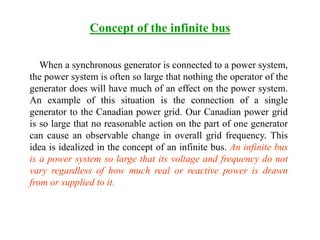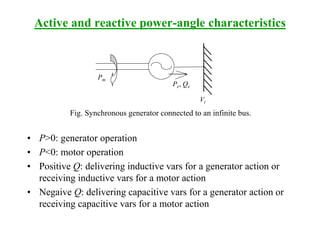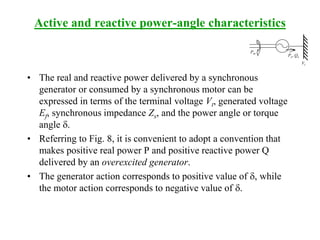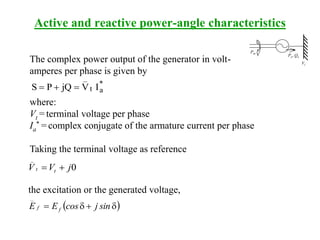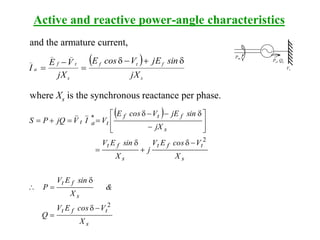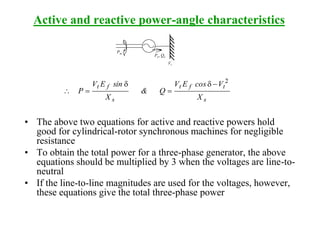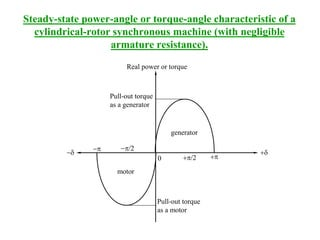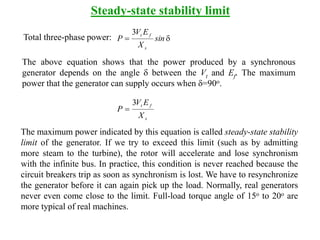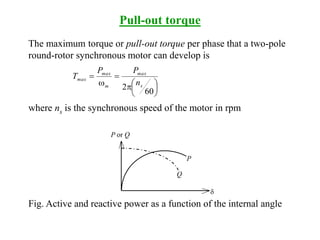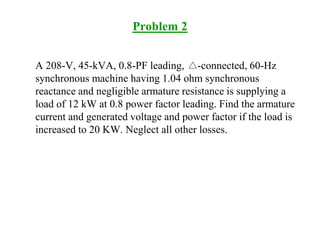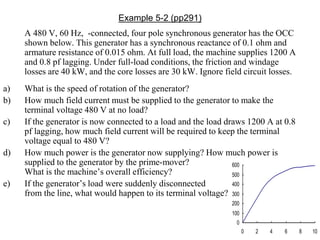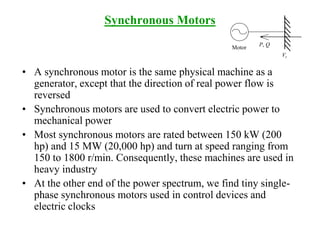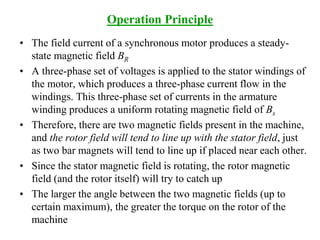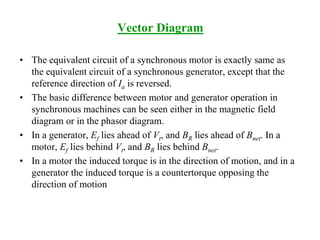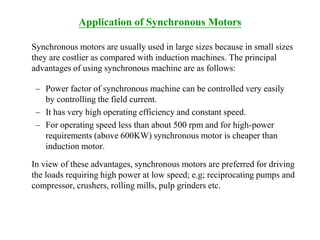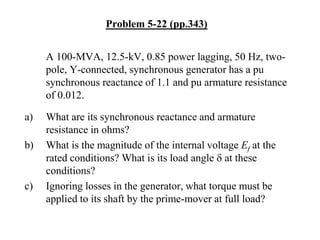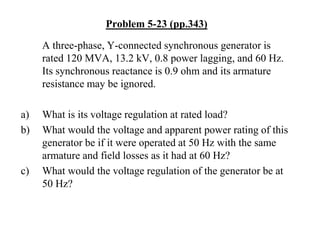1 of 47

### Lectures synchronous machines(1)

• 2. Synchronous Machines • Synchronous generators or alternators are used to convert mechanical power derived from steam, gas, or hydraulic-turbine to ac electric power • Synchronous generators are the primary source of electrical energy we consume today • Large ac power networks rely almost exclusively on synchronous generators • Synchronous motors are built in large units compare to induction motors (Induction motors are cheaper for smaller ratings) and used for constant speed industrial drives
• 3. Construction  Basic parts of a synchronous generator: • Rotor - dc excited winding • Stator - 3-phase winding in which the ac emf is generated  The manner in which the active parts of a synchronous machine are cooled determines its overall physical size and structure
• 4. Various Types  Salient-pole synchronous machine  Cylindrical or round-rotor synchronous machine
• 5. 1. Most hydraulic turbines have to turn at low speeds (between 50 and 300 r/min) 2. A large number of poles are required on the rotor Hydrogenerator Turbine Hydro (water) D  10 m Non-uniform air-gap N S S N d-axis q-axis Salient-Pole Synchronous Generator
• 7. L  10 m D  1 mTurbine Steam Stator Uniform air-gap Stator winding Rotor Rotor winding N S  High speed  3600 r/min  2-pole  1800 r/min  4-pole  Direct-conductor cooling (using hydrogen or water as coolant)  Rating up to 2000 MVA Turbogenerator d-axis q-axis Cylindrical-Rotor Synchronous Generator
• 9. Operation Principle The rotor of the generator is driven by a prime-mover A dc current is flowing in the rotor winding which produces a rotating magnetic field within the machine The rotating magnetic field induces a three-phase voltage in the stator winding of the generator
• 10. Electrical Frequency Electrical frequency produced is locked or synchronized to the mechanical speed of rotation of a synchronous generator: where fe = electrical frequency in Hz P = number of poles nm= mechanical speed of the rotor, in r/min 120 m e nP f 
• 11. Generated Voltage The generated voltage of a synchronous generator is given by where f = flux in the machine (function of If) fe = electrical frequency Kc= synchronous machine constant Saturation characteristic of a synchronous generator. ec fKE f If E
• 12. Voltage Regulation A convenient way to compare the voltage behaviour of two generators is by their voltage regulation (VR). The VR of a synchronous generator at a given load, power factor, and at rated speed is defined as % V VE VR fl flnl 100   Where Vfl is the full-load terminal voltage, and Enl (equal to Ef) is the no-load terminal voltage (internal voltage) at rated speed when the load is removed without changing the field current. For lagging power factor (PF), VR is fairly positive, for unity PF, VR is small positive and for leading PF, VR is negative.
• 13. Equivalent Circuit_1 o The internal voltage Ef produced in a machine is not usually the voltage that appears at the terminals of the generator. o The only time Ef is same as the output voltage of a phase is when there is no armature current flowing in the machine. o There are a number of factors that cause the difference between Ef and Vt: – The distortion of the air-gap magnetic field by the current flowing in the stator, called the armature reaction – The self-inductance of the armature coils. – The resistance of the armature coils. – The effect of salient-pole rotor shapes.
• 14. generator motor Ia Ia Ef Eres Vt jX jXl Ra + + + Equivalent Circuit_2 Equivalent circuit of a cylindrical-rotor synchronous machine
• 15. Phasor Diagram Phasor diagram of a cylindrical-rotor synchronous generator, for the case of lagging power factor Lagging PF: |Vt|<|Ef| for overexcited condition Leading PF: |Vt|>|Ef| for underexcited condition
• 16. Three-phase equivalent circuit of a cylindrical-rotor synchronous machine The voltages and currents of the three phases are 120o apart in angle, but otherwise the three phases are identical. + Ia1 Ef1 jXs Ra+ VL-L VL-L =3Vt Vt
• 17. Determination of the parameters of the equivalent circuit from test data • The equivalent circuit of a synchronous generator that has been derived contains three quantities that must be determined in order to completely describe the behaviour of a real synchronous generator: – The saturation characteristic: relationship between If and f (and therefore between If and Ef) – The synchronous reactance, Xs – The armature resistance, Ra • • The above three quantities could be determined by performing the following three tests: – Open-circuit test – Short-circuit test – DC test
• 18. Open-circuit test • The generator is turned at the rated speed • The terminals are disconnected from all loads, and the field current is set to zero. • Then the field current is gradually increased in steps, and the terminal voltage is measured at each step along the way. • It is thus possible to obtain an open-circuit characteristic of a generator (Ef or Vt versus If) from this information + Vdc If Vt
• 19. Short-circuit test • Adjust the field current to zero and short-circuit the terminals of the generator through a set of ammeters. • Record the armature current Isc as the field current is increased. • Such a plot is called short-circuit characteristic. A A+ Vdc If Isc
• 20. – then – If the stator is Y-connected, the per phase stator resistance is – If the stator is delta-connected, the per phase stator resistance is DC Test – The purpose of the DC test is to determine Ra. A variable DC voltage source is connected between two stator terminals. – The DC source is adjusted to provide approximately rated stator current, and the resistance between the two stator leads is determined from the voltmeter and ammeter readings DC DC DC V R I  2 DC a R R  DCa RR 2 3 
• 21. Determination of Xs • For a particular field current IfA, the internal voltage Ef (=VA) could be found from the occ and the short-circuit current flow Isc,A could be found from the scc. • Then the synchronous reactance Xs could be obtained using IfA Ef or Vt (V) Air-gap line OCC Isc (A) SCC If (A) Vrated VA Isc,B Isc, A IfB   scA fA unsat,saunsat,s I EV XRZ   22 22 aunsat,sunsat,s RZX  scA oc,t scA f unsat,s I V I E X  : Ra is known from the DC test. Since Xs,unsat>>Ra,
• 22. Xs under saturated condition Ia Ef Vt=0 jXs Ra + + EfVt=0 jIaXs IaRa Ia   scB frated sat,sasat,s I EV XRZ   22 At V = Vrated, 22 asat,ssat,s RZX  : Ra is known from the DC test. Equivalent circuit and phasor diagram under condition IfA Ef or Vt (V) Air-gap line OCC Isc (A) SCC If (A) Vrated VA Isc,B Isc, A IfB
• 23. Short-circuit Ratio Another parameter used to describe synchronous generators is the short-circuit ratio (SCR). The SCR of a generator defined as the ratio of the field current required for the rated voltage at open circuit to the field current required for the rated armature current at short circuit. SCR is just the reciprocal of the per unit value of the saturated synchronous reactance calculated by  .u.pinX I I SCR sat_s Iscrated_f Vrated_f 1   Ef or Vt (V) Air-gap line OCC Isc (A) SCC If (A) Vrated Isc,rated If_V rated If_Isc rated
• 24. Example 1 A 200 kVA, 480-V, 60-Hz, 4-pole, Y-Connected synchronous generator with a rated field current of 5 A was tested and the following data was taken. a) from OC test – terminal voltage = 540 V at rated field current b) from SC test – line current = 300A at rated field current c) from Dc test – DC voltage of 10 V applied to two terminals, a current of 25 A was measured. 1. Calculate the speed of rotation in r/min 2. Calculate the generated emf and saturated equivalent circuit parameters (armature resistance and synchronous reactance)
• 25. Solution to Example 1 1. fe = electrical frequency = Pnm/120 fe = 60Hz P = number of poles = 4 nm = mechanical speed of rotation in r/min. So, speed of rotation nm = 120 fe / P = (120 x 60)/4 = 1800 r/min 2. In open-circuit test, Ia = 0 and Ef =Vt Ef = 540/1.732 = 311.8 V (as the machine is Y-connected) In short-circuit test, terminals are shorted, Vt = 0 Ef = IaZs or Zs = Ef /Ia =311.8/300=1.04 ohm From the DC test, Ra=VDC/(2IDC) = 10/(2X25) = 0.2 ohm Synchronous reactance 2 , 2 , satsasats XRZ  02.12.004.1 2222 ,,  asatssats RZX Ia Ef Vt j1.02 0.2 + +
• 26. Problem 1 A 480-V, 60-Hz, Y-Connected synchronous generator, having the synchronous reactance of 1.04 ohm and negligible armature resistance, is operating alone. The terminal voltage at rated field current at open circuit condition is 480V. 1. Calculate the voltage regulation 1. If load current is 100A at 0.8 PF lagging 2. If load current is 100A at 0.8 PF leading 3. If load current is 100A at unity PF 2. Calculate the real and reactive power delivered in each case. 3. State and explain whether the voltage regulation will improve or not if the load current is decreased to 50 A from 100 A at 0.8 PF lagging.
• 27. Parallel operation of synchronous generators There are several major advantages to operate generators in parallel: • Several generators can supply a bigger load than one machine by itself. • Having many generators increases the reliability of the power system. • It allows one or more generators to be removed for shutdown or preventive maintenance.
• 28. Before connecting a generator in parallel with another generator, it must be synchronized. A generator is said to be synchronized when it meets all the following conditions: • The rms line voltages of the two generators must be equal. • The two generators must have the same phase sequence. • The phase angles of the two a phases must be equal. • The oncoming generator frequency is equal to the running system frequency. Synchronization Load Generator 2 Generator 1 Switch a b c a/ b/ c/
• 29. Synchronization LoadGenerator Rest of the power system Generator Xs1 Ef1 Xs2 Ef2 Xsn Efn Infinite bus V, f are constant Xs eq = 0 G
• 30. Concept of the infinite bus When a synchronous generator is connected to a power system, the power system is often so large that nothing the operator of the generator does will have much of an effect on the power system. An example of this situation is the connection of a single generator to the Canadian power grid. Our Canadian power grid is so large that no reasonable action on the part of one generator can cause an observable change in overall grid frequency. This idea is idealized in the concept of an infinite bus. An infinite bus is a power system so large that its voltage and frequency do not vary regardless of how much real or reactive power is drawn from or supplied to it.
• 31. Active and reactive power-angle characteristics • P>0: generator operation • P<0: motor operation • Positive Q: delivering inductive vars for a generator action or receiving inductive vars for a motor action • Negaive Q: delivering capacitive vars for a generator action or receiving capacitive vars for a motor action Pm Pe, Qe Vt Fig. Synchronous generator connected to an infinite bus.
• 32. Active and reactive power-angle characteristics • The real and reactive power delivered by a synchronous generator or consumed by a synchronous motor can be expressed in terms of the terminal voltage Vt, generated voltage Ef, synchronous impedance Zs, and the power angle or torque angle d. • Referring to Fig. 8, it is convenient to adopt a convention that makes positive real power P and positive reactive power Q delivered by an overexcited generator. • The generator action corresponds to positive value of d, while the motor action corresponds to negative value of d. Pm Pe, Qe Vt
• 33. The complex power output of the generator in volt- amperes per phase is given by * at _ IVjQPS  where: Vt = terminal voltage per phase Ia * = complex conjugate of the armature current per phase Taking the terminal voltage as reference 0jVV tt _  the excitation or the generated voltage,  dd sinjcosEE ff _ Active and reactive power-angle characteristics Pm Pe, Qe Vt
• 34. Active and reactive power-angle characteristics Pm Pe, Qe Vt and the armature current,   s ftf s t _ f _ a _ jX sinjEVcosE jX VE I dd    where Xs is the synchronous reactance per phase.   s tft s ft s tft s ft s ftf t * a _ t _ X VcosEV Q & X sinEV P X VcosEV j X sinEV jX sinjEVcosE VIVjQPS 2 2 d  d  d  d           dd 
• 35. Active and reactive power-angle characteristics Pm Pe, Qe Vt s tft s ft X VcosEV Q& X sinEV P 2 d  d  • The above two equations for active and reactive powers hold good for cylindrical-rotor synchronous machines for negligible resistance • To obtain the total power for a three-phase generator, the above equations should be multiplied by 3 when the voltages are line-to- neutral • If the line-to-line magnitudes are used for the voltages, however, these equations give the total three-phase power
• 36. Steady-state power-angle or torque-angle characteristic of a cylindrical-rotor synchronous machine (with negligible armature resistance). d Real power or torque generator motor pp/2 p/2 0 p Pull-out torque as a generator Pull-out torque as a motor d
• 37. Steady-state stability limit Total three-phase power: d sin X EV P s ft3 The above equation shows that the power produced by a synchronous generator depends on the angle d between the Vt and Ef. The maximum power that the generator can supply occurs when d=90o. s ft X EV P 3  The maximum power indicated by this equation is called steady-state stability limit of the generator. If we try to exceed this limit (such as by admitting more steam to the turbine), the rotor will accelerate and lose synchronism with the infinite bus. In practice, this condition is never reached because the circuit breakers trip as soon as synchronism is lost. We have to resynchronize the generator before it can again pick up the load. Normally, real generators never even come close to the limit. Full-load torque angle of 15o to 20o are more typical of real machines.
• 38. Pull-out torque The maximum torque or pull-out torque per phase that a two-pole round-rotor synchronous motor can develop is      p    60 2 s max m max max n PP T where ns is the synchronous speed of the motor in rpm P d P or Q Q Fig. Active and reactive power as a function of the internal angle
• 39. Problem 2 A 208-V, 45-kVA, 0.8-PF leading, -connected, 60-Hz synchronous machine having 1.04 ohm synchronous reactance and negligible armature resistance is supplying a load of 12 kW at 0.8 power factor leading. Find the armature current and generated voltage and power factor if the load is increased to 20 KW. Neglect all other losses.
• 40. Example 5-2 (pp291) A 480 V, 60 Hz, -connected, four pole synchronous generator has the OCC shown below. This generator has a synchronous reactance of 0.1 ohm and armature resistance of 0.015 ohm. At full load, the machine supplies 1200 A and 0.8 pf lagging. Under full-load conditions, the friction and windage losses are 40 kW, and the core losses are 30 kW. Ignore field circuit losses. a) What is the speed of rotation of the generator? b) How much field current must be supplied to the generator to make the terminal voltage 480 V at no load? c) If the generator is now connected to a load and the load draws 1200 A at 0.8 pf lagging, how much field current will be required to keep the terminal voltage equal to 480 V? d) How much power is the generator now supplying? How much power is supplied to the generator by the prime-mover? What is the machine’s overall efficiency? e) If the generator’s load were suddenly disconnected from the line, what would happen to its terminal voltage? 0 100 200 300 400 500 600 0 2 4 6 8 10
• 41. Synchronous Motors • A synchronous motor is the same physical machine as a generator, except that the direction of real power flow is reversed • Synchronous motors are used to convert electric power to mechanical power • Most synchronous motors are rated between 150 kW (200 hp) and 15 MW (20,000 hp) and turn at speed ranging from 150 to 1800 r/min. Consequently, these machines are used in heavy industry • At the other end of the power spectrum, we find tiny single- phase synchronous motors used in control devices and electric clocks P, Q Vt Motor
• 42. Operation Principle • The field current of a synchronous motor produces a steady- state magnetic field BR • A three-phase set of voltages is applied to the stator windings of the motor, which produces a three-phase current flow in the windings. This three-phase set of currents in the armature winding produces a uniform rotating magnetic field of Bs • Therefore, there are two magnetic fields present in the machine, and the rotor field will tend to line up with the stator field, just as two bar magnets will tend to line up if placed near each other. • Since the stator magnetic field is rotating, the rotor magnetic field (and the rotor itself) will try to catch up • The larger the angle between the two magnetic fields (up to certain maximum), the greater the torque on the rotor of the machine
• 43. Vector Diagram • The equivalent circuit of a synchronous motor is exactly same as the equivalent circuit of a synchronous generator, except that the reference direction of Ia is reversed. • The basic difference between motor and generator operation in synchronous machines can be seen either in the magnetic field diagram or in the phasor diagram. • In a generator, Ef lies ahead of Vt, and BR lies ahead of Bnet. In a motor, Ef lies behind Vt, and BR lies behind Bnet. • In a motor the induced torque is in the direction of motion, and in a generator the induced torque is a countertorque opposing the direction of motion
• 44. Vector Diagram d Ia Vt Ef jIa Xs d Ia Vt Ef jIa Xs d Bs Bnet BR sync Fig. The phasor diagram (leading PF: overexcited and |Vt|<|Ef|) and the corresponding magnetic field diagram of a synchronous motor. Fig. The phasor diagram of an underexcited synchronous motor (lagging PF and |Vt|>|Ef|).
• 45. Application of Synchronous Motors Synchronous motors are usually used in large sizes because in small sizes they are costlier as compared with induction machines. The principal advantages of using synchronous machine are as follows: – Power factor of synchronous machine can be controlled very easily by controlling the field current. – It has very high operating efficiency and constant speed. – For operating speed less than about 500 rpm and for high-power requirements (above 600KW) synchronous motor is cheaper than induction motor. In view of these advantages, synchronous motors are preferred for driving the loads requiring high power at low speed; e.g; reciprocating pumps and compressor, crushers, rolling mills, pulp grinders etc.
• 46. Problem 5-22 (pp.343) A 100-MVA, 12.5-kV, 0.85 power lagging, 50 Hz, two- pole, Y-connected, synchronous generator has a pu synchronous reactance of 1.1 and pu armature resistance of 0.012. a) What are its synchronous reactance and armature resistance in ohms? b) What is the magnitude of the internal voltage Ef at the rated conditions? What is its load angle d at these conditions? c) Ignoring losses in the generator, what torque must be applied to its shaft by the prime-mover at full load?
• 47. Problem 5-23 (pp.343) A three-phase, Y-connected synchronous generator is rated 120 MVA, 13.2 kV, 0.8 power lagging, and 60 Hz. Its synchronous reactance is 0.9 ohm and its armature resistance may be ignored. a) What is its voltage regulation at rated load? b) What would the voltage and apparent power rating of this generator be if it were operated at 50 Hz with the same armature and field losses as it had at 60 Hz? c) What would the voltage regulation of the generator be at 50 Hz?